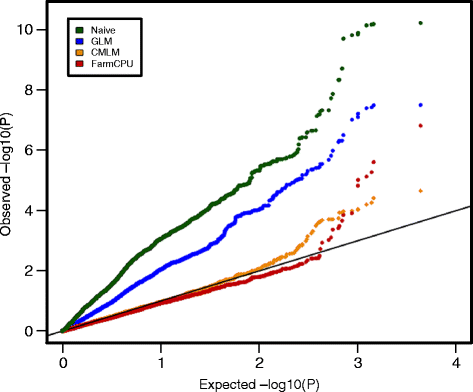How to make Q-Q plots for different models at a single chart using R?
1
0
Entering edit mode
20 months ago
rimgubaev ▴ 220

Hello everyone! I am trying to make a Q-Q plot to visualise p-values obtained from different models afterr runing GWAS analysis. The key thing is that I want to reflect p-values from the different models in one chart. I tried qq function in qqman package however it takes only a vector of one model as an input, so as a result, I get the following picture:And what I am trying to should look like this:GWAS qqman Q–Q plot model selection • 2.7k views
0
Entering edit mode

I don't understand the desired plot. The QQ plot use the mean and variance of the vector you inserted to plot it against a normal distribution, I don't see how you can compare different vectors with different means and variances. Having said that, you can use the returned values from qqnorm to plot multiple vectors in one figure.

0
Entering edit mode

For normal distribution it is always possible to do standartization (Normal => Standard Normal), so this is not a big problem. QQ plot does not require the distribution to be normal - sample quantiles of, e.g., exponential distribution, may be plotted against theoretical quantiles (https://stat.ethz.ch/R-manual/R-devel/library/stats/html/qqnorm.html , there is an example of chisq at the bottom).

y <- rchisq(500, df = 3)
z <- rchisq(500, df = 3)
## Q-Q plot for Chi^2 data against true theoretical distribution:
vals1 <- qqplot(qchisq(ppoints(500), df = 3), y,
main = expression("Q-Q plot for" ~~ {chi^2}[nu == 3]))
vals = qqplot(qchisq(ppoints(500), df = 3), z,
main = expression("Q-Q plot for" ~~ {chi^2}[nu == 3]))
plot(vals1$x, vals1$y, pch=19, col="black")
points(vals$x, vals$y, pch=19, col="red")
abline(a=0, b=1)

4
Entering edit mode
20 months ago
Lemire ▴ 720

You could plot it outside any qq plotting functions. The following will generate the same graph:

Generate p-values:

p<- runif( 10000 )


Using qqplot:

qqplot( -log10( ppoints(p ) ), -log10( p ) , col="red" )


Using plot:

p.sorted <- sort(p)
plot( -log10(ppoints(p.sorted )), -log10(p.sorted) , col="red")


(same as above). Then if you want to add another data set:

p2<- runif(5000)
p2.sorted <- sort(p2)
points( -log10(ppoints(p2.sorted )), -log10(p2.sorted) , col="blue" )


You may have to play with the xlim and ylim argument of the plot function to include all values of subsequent datasets.

0
Entering edit mode

It is perfect! But how to add a line to aplot like in the qqman example above?

1
Entering edit mode

With

abline( 0,1 )